1594102200

# Introduction

In this article, we will analyse a business problem with linear regression in a step by step manner and try to interpret the statistical terms at each step to understand its inner workings. Although the liner regression algorithm is simple, for proper analysis, one should interpret the statistical results.

First, we will take a look at simple linear regression and after extending the problem to multiple linear regression.

For easy understanding, follow the python notebook side by side.

# What is Linear Regression?

Regression is the statistical approach to find the relationship between variables. Hence, the** Linear Regression** assumes a linear relationship between variables. Depending on the number of input variables, the regression problem classified into

1. Simple linear regression

2. Multiple linear regression

Let’s consider there is a company and it has to improve the sales of the product. The company spends money on different advertising media such as TV, radio, and newspaper to increase the sales of its products. The company records the money spent on each advertising media (in thousands of dollars) and the number of units of product sold (in thousands of units).

Now we have to help the company to find out the most effective way to spend money on advertising media to improve sales for the next year with a less advertising budget.

# Simple Linear Regression

Simple linear is an approach for predicting the quantitative response Y based on single predictor variable X.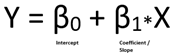This is the equation of straight-line having slope β1 and intercept β0.

Let’s start the regression analysis for given advertisement data with simple linear regression. Initially, we will consider the simple linear regression model for the sales and money spent on TV advertising media.

Then the mathematical equation becomes 𝑆𝑎𝑙𝑒𝑠 = 𝛽0 + 𝛽1 * 𝑇𝑉.

## Step 1: Estimating the coefficients: (Let’s find the coefficients)

Now to find the estimate of the sales for the advertising budget, we have to know the values of the β1 and β0. For the best estimate, the difference between predicted sales and the actual sales (called as residual) should be minimum.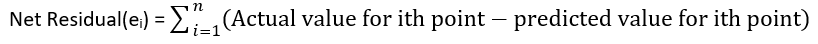As the residual may be negative or positive, so while calculating the net residual it can be lead to cancellation terms and reduction of net effect which leads to a non-optimal estimate of coefficients. To overcome this, we use a Residual sum of squares (RSS).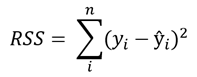With a simple calculation, we can find the value of β0 and β1 for minimum RSS value.

With the stats model library in python, we can find out the coefficients,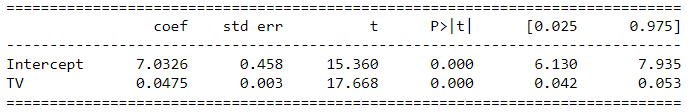Table 1: Simple regression of sales on TV

Values for β0 and β1 are 7.03 and 0.047 respectively. Then the relation becomes, Sales = 7.03 + 0.047 * TV.

This means if we spend an additional 1000 dollars on TV advertising media it increases the sales of products by 47 units.

This gives us how strongly the TV advertising media associated with the sales.

## Step 2: Assessing the Accuracy of the Coefficient Estimates ( How accurate these coefficients are? )

Why the coefficients are not perfect estimates?

The true relationship may not be perfectly linear, so there is an error that can be reduced by using a more complex model such as the polynomial regression model. These types of errors are called reducible errors.

On the other hand, errors may introduce because of errors in measurement and environmental conditions such as the office is closed for one week due to heavy rain which affects the sales. These types of errors are called**_ irreducible errors_**.

#linear-regression #machine-learning #basics #regression-analysis #data-science #data analysis

## Buddha Community1594102200

# Introduction

In this article, we will analyse a business problem with linear regression in a step by step manner and try to interpret the statistical terms at each step to understand its inner workings. Although the liner regression algorithm is simple, for proper analysis, one should interpret the statistical results.

First, we will take a look at simple linear regression and after extending the problem to multiple linear regression.

For easy understanding, follow the python notebook side by side.

# What is Linear Regression?

Regression is the statistical approach to find the relationship between variables. Hence, the** Linear Regression** assumes a linear relationship between variables. Depending on the number of input variables, the regression problem classified into

1. Simple linear regression

2. Multiple linear regression

Let’s consider there is a company and it has to improve the sales of the product. The company spends money on different advertising media such as TV, radio, and newspaper to increase the sales of its products. The company records the money spent on each advertising media (in thousands of dollars) and the number of units of product sold (in thousands of units).

Now we have to help the company to find out the most effective way to spend money on advertising media to improve sales for the next year with a less advertising budget.

# Simple Linear Regression

Simple linear is an approach for predicting the quantitative response Y based on single predictor variable X.This is the equation of straight-line having slope β1 and intercept β0.

Let’s start the regression analysis for given advertisement data with simple linear regression. Initially, we will consider the simple linear regression model for the sales and money spent on TV advertising media.

Then the mathematical equation becomes 𝑆𝑎𝑙𝑒𝑠 = 𝛽0 + 𝛽1 * 𝑇𝑉.

## Step 1: Estimating the coefficients: (Let’s find the coefficients)

Now to find the estimate of the sales for the advertising budget, we have to know the values of the β1 and β0. For the best estimate, the difference between predicted sales and the actual sales (called as residual) should be minimum.As the residual may be negative or positive, so while calculating the net residual it can be lead to cancellation terms and reduction of net effect which leads to a non-optimal estimate of coefficients. To overcome this, we use a Residual sum of squares (RSS).With a simple calculation, we can find the value of β0 and β1 for minimum RSS value.

With the stats model library in python, we can find out the coefficients,Table 1: Simple regression of sales on TV

Values for β0 and β1 are 7.03 and 0.047 respectively. Then the relation becomes, Sales = 7.03 + 0.047 * TV.

This means if we spend an additional 1000 dollars on TV advertising media it increases the sales of products by 47 units.

This gives us how strongly the TV advertising media associated with the sales.

## Step 2: Assessing the Accuracy of the Coefficient Estimates ( How accurate these coefficients are? )

Why the coefficients are not perfect estimates?

The true relationship may not be perfectly linear, so there is an error that can be reduced by using a more complex model such as the polynomial regression model. These types of errors are called reducible errors.

On the other hand, errors may introduce because of errors in measurement and environmental conditions such as the office is closed for one week due to heavy rain which affects the sales. These types of errors are called**_ irreducible errors_**.

#linear-regression #machine-learning #basics #regression-analysis #data-science #data analysis1594271340

## A Deep Dive into Linear Regression

Let’s begin our journey with the truth — machines never learn. What a typical machine learning algorithm does is find a mathematical equation that, when applied to a given set of training data, produces a prediction that is very close to the actual output.

Why is this not learning? Because if you change the training data or environment even slightly, the algorithm will go haywire! Not how learning works in humans. If you learned to play a video game by looking straight at the screen, you would still be a good player if the screen is slightly tilted by someone, which would not be the case in ML algorithms.

However, most of the algorithms are so complex and intimidating that it gives our mere human intelligence the feel of actual learning, effectively hiding the underlying math within. There goes a dictum that if you can implement the algorithm, you know the algorithm. This saying is lost in the dense jungle of libraries and inbuilt modules which programming languages provide, reducing us to regular programmers calling an API and strengthening further this notion of a black box. Our quest will be to unravel the mysteries of this so-called ‘black box’ which magically produces accurate predictions, detects objects, diagnoses diseases and claims to surpass human intelligence one day.

We will start with one of the not-so-complex and easy to visualize algorithm in the ML paradigm — Linear Regression. The article is divided into the following sections:

1. Need for Linear Regression

2. Visualizing Linear Regression

3. Deriving the formula for weight matrix W

4. Using the formula and performing linear regression on a real world data set

Note: Knowledge on Linear Algebra, a little bit of Calculus and Matrices are a prerequisite to understanding this article

Also, a basic understanding of python, NumPy, and Matplotlib are a must.

## 1) Need for Linear regression

Regression means predicting a real valued number from a given set of input variables. Eg. Predicting temperature based on month of the year, humidity, altitude above sea level, etc. Linear Regression would therefore mean predicting a real valued number that follows a linear trend. Linear regression is the first line of attack to discover correlations in our data.

Now, the first thing that comes to our mind when we hear the word linear is, a line.

Yes! In linear regression, we try to fit a line that best generalizes all the data points in the data set. By generalizing, we mean we try to fit a line that passes very close to all the data points.

But how do we ensure that this happens? To understand this, let’s visualize a 1-D Linear Regression. This is also called as Simple Linear Regression

#calculus #machine-learning #linear-regression-math #linear-regression #linear-regression-python #python1603033200

## A Complete Guide to Linear Regression for Beginners

What is supervised learning? In supervised learning, you have an input-output pair. And you will try to map the given input to output by training the input-output pair.

Another type of machine learning algorithm is unsupervised learning, in this, you don’t have an output variable. You will try to group the input variables by their similarities.

What is Regression? Regression is a statistical process of estimating the relationship between a dependant variable and several independent variables.

In other words, it can be said that linear regression suggests that the output variable can be represented as a linear combination of the input variables.

Linear Regression Example

Depending upon the number of input variables, linear regression can be classified into simple and multiple linear regression. If the number of input variables is one, then it is called simple linear regression.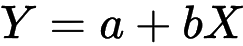Simple Linear Regression Formula

If there is more than one input variable, then it is called multiple linear regression.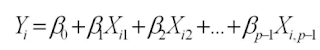Multiple Linear Regression Formula

In this blog post, I will be discussing simple linear regression.1598352300

## Regression: Linear Regression

Machine learning algorithms are not your regular algorithms that we may be used to because they are often described by a combination of some complex statistics and mathematics. Since it is very important to understand the background of any algorithm you want to implement, this could pose a challenge to people with a non-mathematical background as the maths can sap your motivation by slowing you down.In this article, we would be discussing linear and logistic regression and some regression techniques assuming we all have heard or even learnt about the Linear model in Mathematics class at high school. Hopefully, at the end of the article, the concept would be clearer.

**Regression Analysis **is a statistical process for estimating the relationships between the dependent variables (say Y) and one or more independent variables or predictors (X). It explains the changes in the dependent variables with respect to changes in select predictors. Some major uses for regression analysis are in determining the strength of predictors, forecasting an effect, and trend forecasting. It finds the significant relationship between variables and the impact of predictors on dependent variables. In regression, we fit a curve/line (regression/best fit line) to the data points, such that the differences between the distances of data points from the curve/line are minimized.

#regression #machine-learning #beginner #logistic-regression #linear-regression #deep learning1592023980

## 5 Regression algorithms: Explanation & Implementation in Python

Take your current understanding and skills on machine learning algorithms to the next level with this article. What is regression analysis in simple words? How is it applied in practice for real-world problems? And what is the possible snippet of codes in Python you can use for implementation regression algorithms for various objectives? Let’s forget about boring learning stuff and talk about science and the way it works.

#linear-regression-python #linear-regression #multivariate-regression #regression #python-programming